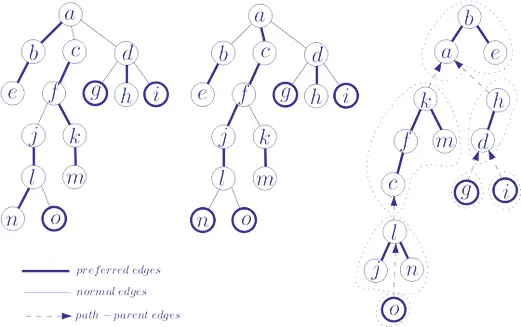## Data Structure Tutorial Index

In a computer application, you usually do not need to study trees in such generality, and when you do, for emphasis you call them as free trees. The trees you create are almost always tied down by having one particular vertex singled out as the root or for emphasis you can call such trees as a rooted tree.

In a rooted tree, there is still no way to tell left from right or when one vertex has several children, to tell which is first, second and so on. So for no other reasons, the restraint of sequential execution of instructions (not to the mentioned sequential organization of storage) usually imposes an order on the children of each vertex. Hence you can define an ordered tree to be a rooted tree in which children o each vertex are assigned an order.

In this chapter you will learn about the basic concepts of forests and how orchards are formed in data structure.

## What are Forests and Orchards?

In your understanding so far with binary trees you have got benefits for using binary tree. Binary trees can also be implemented using recursion which reduces a problem to a smaller one.

There is a standard term for an arbitrary set of trees which is called a forest. In other words, you can say a general tree as the root of a forest, and a forest is an ordered combination of zero or more general trees. This mutually recursive definition of general trees and forests allows programmers to utter about trees where nodes may have more than two children. These children of a node (the trees of the forest that it roots) are sequenced: the first, the second, and so on. There is no concept of left and right in general trees, except you can typically draw the tree with the sequential ordering from left to right.When you are using the term forest, you will generally assume that the tree is rooted. The phrase ordered forest is sometimes used for an ordered set of ordered trees, but you will adopt the equally descriptive term orchards. You can also say that the standard term for an arbitrary set of trees is a forest. It is to be noted that you can only obtain a forest or an orchard by removing the rot from a rooted tree - by starting with a forest or an orchard, attaching a new vertex on the top and adding branches from the new vertex to the root of all trees I forest or an orchard.

## What is Rotation?

Rotation is the transformation from orchards to a binary tree. In a binary tree [v, f(O1), f(O2)] of the left link from v goes to the root of the binary tree f(O1), which in fact is the first child of V in the ordered tree {V, O1}. In geometrical terms, the transformation reduces to the following rules explained below -

• Draw the orchard so that the first child of each vertex is immediately below the vertex.
• Draw a vertical link from each vertex to its first child.
• Draw a horizontal link from each vertex to its next sibling.
• Remove those remaining original links
• Rotate the diagram at an angle of 45o (degree) clockwise which appear as a left link and horizontal links appear as the right one.